# Two xeroxes

The performances of the two copiers are in the ratio 3: 4. A machine with higher power will make 7,200 copies in one hour. How many copies will both machines make together in 5 hours?

Correct result:

s =  63000

#### Solution: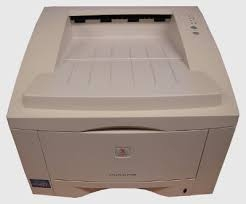We would be pleased if you find an error in the word problem, spelling mistakes, or inaccuracies and send it to us. Thank you!Tips to related online calculators
Need help calculate sum, simplify or multiply fractions? Try our fraction calculator.
Check out our ratio calculator.
Do you want to convert time units like minutes to seconds?

## Next similar math problems:

• Three machines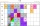The power of the three machines is 2: 3: 5. Two most powerful machines produce 400 parts per hour. How many components make all three machines in 3 hours?
• Two machinesPerformances of two machines are in a ratio of 7:12. A machine with less power produced 406 pieces of products per shift. a) How many pieces produced per shift second machine? b) How many pieces produced two machines together for five shifts?
• Two pumps togetherThe first pump will fill the tank itself in 3 hours and the second one in 6 hours. How many hours will the tank be full if both pumps are worked at the same time?
• Two looms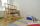On two different looms with different performance can be make required quantity of textile in 6 hours when both work together. On the first machine this quantity of the textile can make in 10 hours. How long should take make same quantity of textile on a
• Master and apprentice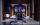Master painted the roof in 3 hours and apprentice for 4 hours. How many of roof they painted in hour and how many in three quarters of an hour?
• Two machinesThe first machine can produce parts in 24 hours, the second in 16 hours. The first started working in the morning at 6:00 pm. Second at 10:00 o'clock. At what hour were all parts made?
• Five combers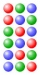Five combers harvest 12 rows of strawberries in 4 hours. How many rows of strawberries will two combers harvest in 10 hours?
• Machines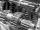In the workshop are three machines. The first is treated 250 parts for 4.7 hours, the second is treated 300 parts for 1.9 hours and the third is treated 230 parts for 4.6 hours. How long will take to make 3100 parts if worked all three machines at the sam
• The machineThe machine works 7 hours a day and produces 1 part in 5 minutes. How many parts will it produce in 1 hour? How many parts will it produce in 1 day?
• Tributaries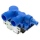The pool can be filled with two different tributaries. The first inflow would fill the pool in 18 hours, both in 6 hours. How many hours would the pool filled with a second inflow?
• Machines 2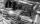2 machines for 50 hours produces 2,000 products. How many machines need to buy to make in 30 hours 15,000 products?
• Copiers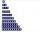Three copiers will do the same in an hour k copies. How many copies would be done in an hour by four such copiers? ?
• Two water containers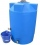In the first container, there are 200 m3 of water and in the second 40 m3. The first container will flow down at a rate of 10 m3 water per hour. At the same time flows to the second rate of 5 m3 per hour. After how many hours there will be three times les
• TogetherGrandfather, father, and son are carpenters. They decided to make a wardrobe. Father produces it for 12 hours, his son for 15 hours, and grandfather for 10 hours. How long will they make it together?
• Work togetherTwo bricklayers plastering a wall. The first would plaster it in 8 hours, the second in 12 hours. How many hours will they be done with the work if they work together?
• Greenhouses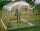Two workers would make installation of greenhouses for 54 hours. For how many hours does the installation 9 workers?
• Together 3If Sally can paint a house in 4 hours, and John can paint the same house in 6 hours, how long will it take for both of them to paint the house together?